# CUMIPMT Function

Calculates the cumulative interest paid on a loan taken or an investment made

## What is the CUMIPMT Function?

The CUMIPMT Function is an Excel Financial function. CUMIPMT helps in calculating the cumulative interest paid on a loan taken out, or earned on an investment made.

Obviously, this function can be helpful in financial analysis, such as in evaluating the return on an investment.

### Formula

=CUMIPMT(rate, nper, pv, start_period, end_period, type)

The CUMIPMT function uses the following arguments:

1. Rate (required argument) – This is the rate of interest per period.
2. Nper (required argument) – The total number of payment periods for which the loan or investment is to be paid.
3. Pv (required argument) – This is the Present Value of the loan/investment.
4. Start_period (required argument) – The number of the first period over which the interest is to be calculated. It needs to be an integer between 1 and the specified NPER.
5. End_period (required argument) – This is the last period over which interest is to be calculated. It must be an integer between 1 and the specified NPER.
6. Type (required argument) – This value can be 0 or 1. It is an integer that specifies if the interest payment is made at the start of the period (0) or the end of the period (1).

### How to use the CUMIPMT Function in Excel?

To understand the uses of the CUMIPMT function, let us consider a few examples:

#### Example 1

Let’s assume we took out a \$100,000 loan that needs to be paid off over 5 years. The interest charged by the bank is 10% and we are required to make monthly payments. First, we need to arrange the data in such a manner that it becomes easier to apply the formula and avoid errors.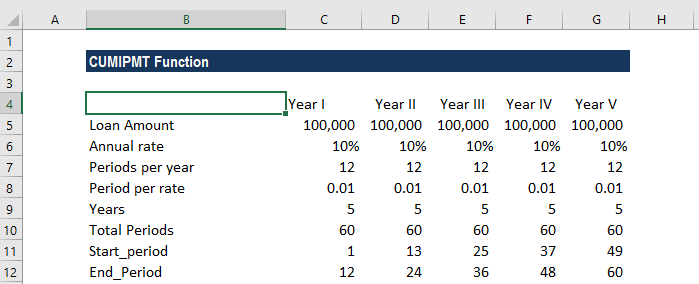We will do this for each year of the loan term. As seen in the screenshot above, the start and end periods change for each year. To avoid errors, we can use a formula. I used the following formula for the start_period: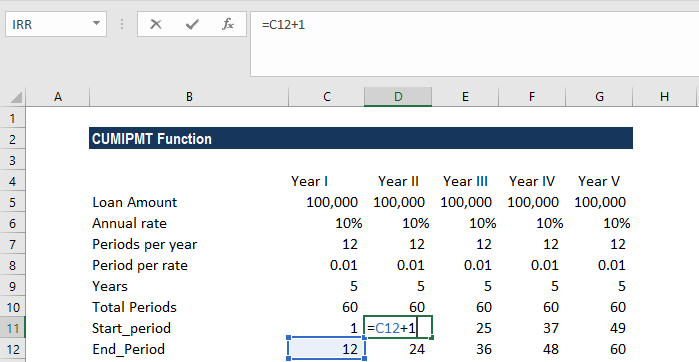For the end_period, I used the following formula: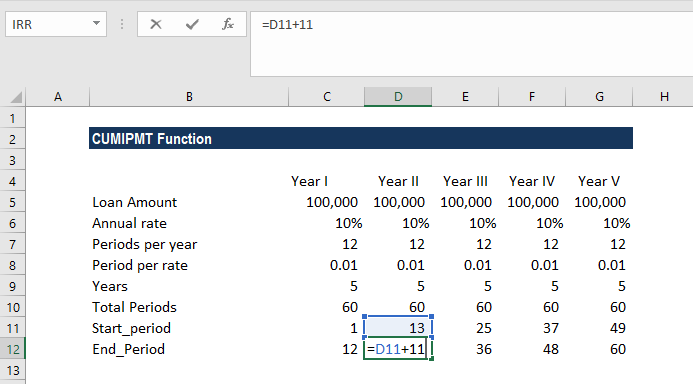As seen above, the interest rate charged is on a per annum basis. We need to convert it to a monthly rate (10%/12). It is the period rate in our table above.

Also, there are 60 total payments, since we are making monthly payments.

Let’s see how this formula can be used in the given scenario: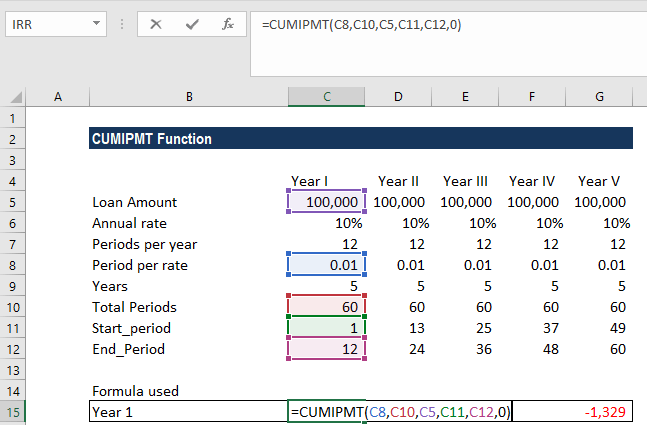We will get the result below: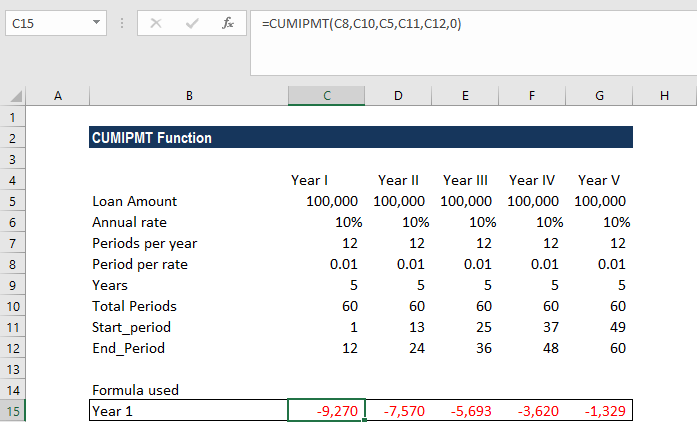The calculated interest payments are negative values, as they represent outgoing payments by the party who took out the loan.

Often, this formula may give very low results. This typically happens when we forget to convert the annual interest rate or the number of periods to months or quarters as required. To avoid errors, we need to make sure that we are consistent about units for specifying rate and nper.

#### Example 2

With the same data as above, let’s see how the results would change if we were required to make quarterly payments. When calculating quarterly payments, the number of periods per year will change to 4.

The table will look like this: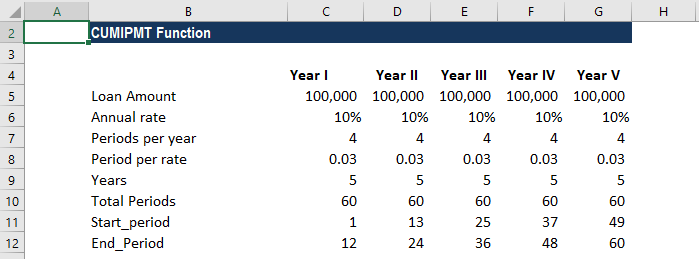The formula to use will be: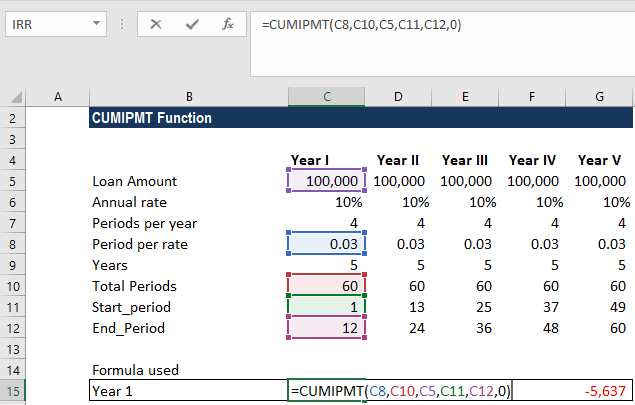We will get the following result: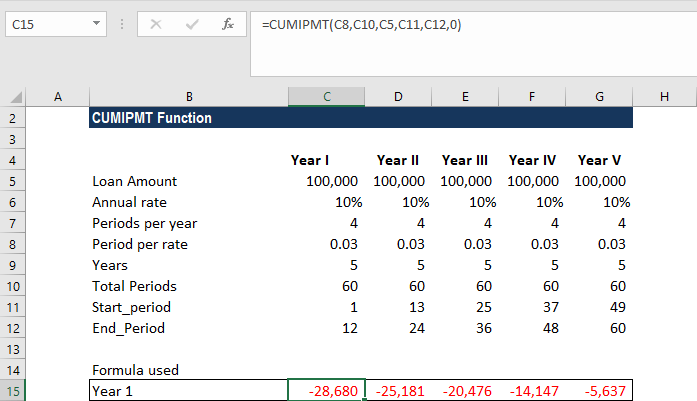### A few things to remember about CUMIPMT

1. #NUM! error – Occurs in the following scenarios:
1. When the given start_period or end_period is less than or equal to zero.
2. When the given start_period is greater than end_period.
3. When any of the given arguments – rate, nper, or PV – is less than or equal to zero.
4. The argument type is not equal to 0 or 1.
2. #VALUE! error – Occurs when any of the given arguments is a non-numeric value or is not recognized as a numerical value by Excel.

Thanks for reading CFI’s guide to important Excel functions! By taking the time to learn and master these functions, you’ll significantly speed up your financial analysis. To learn more, check out these additional CFI resources:

• Excel Functions for Finance